Texas Go Math Grade 2 Unit 1 Answer Key Number and Operations: Place Value, Fraction Concepts, and Addition

Refer to our Texas Go Math Grade 2 Answer Key Pdf to score good marks in the exams. Test yourself by practicing the problems from Texas Go Math Grade 2 Unit 1 Answer Key Number and Operations: Place Value, Fraction Concepts, and Addition.

Texas Go Math Grade 2 Unit 1 Answer Key Number and Operations: Place Value, Fraction Concepts, and Addition

Show What You Know

Check your understanding of important skills.

Identify Numbers to 30.

Write the number that tells how many.

Question 1.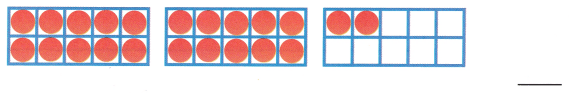Explanation:
In the above-given, figure,
There are 3 groups of boxes.
in group 1 the boxes all are filled.
in group 2 the boxes are filled.
In group 3 only 2 boxes are filled
so in total, there are 22 boxes.
20 + 2 = 22
All the natural numbers are called counting numbers. These numbers are always positive integers like 1,2,3,4,5,6,…… The counting numbers, which can be counted, are infinite and are a crucial part of the number system in Maths.

Count by Ones to 100

Use the hundred chart.

Question 2.
Count from 36 to 47. Which of the numbers below will you say? Circle them.42 31 48 39 37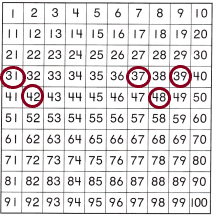Explanation:
In the above-given figure,
Given that 100 charts,
We need to count from 36 to 47.
36, 37, 38, 39, 40, 41, 42, 43, 44, 45, 46, 47.
And we need to circle the given numbers.
Those are 31, 37, 39, 42, 48.

Explore Tens

Write how many tens. Write the number.

Question 3.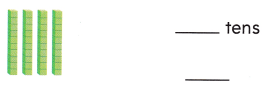Explanation:
There are 4 blocks of tens which are equivalent to each block is 10.
This we can write as 4*10=40.
Therefore, 40 marbles are there overall.

Question 4.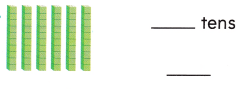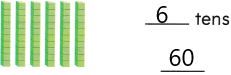Explanation:
There are 10 marbles in each stack.
Likewise, 6 stacks are there.
6 tens are nothing but 6*10=60
Therefore, 60 marbles are there overall.

FAMILY NOTE: This page checks your child’s understanding of important skills needed for success in Unit 1.

Vocabulary Builder

Visualize It
Fill in the boxes of the graphic organizer. Write sentences about ones and tens.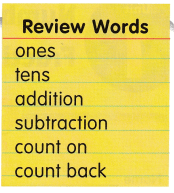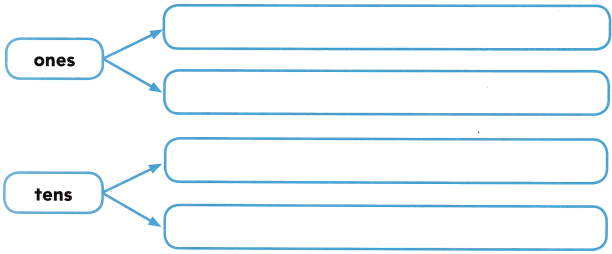Explanation:
Counting numbers are the set of numbers that we use to learn how to count. 1, 2, 3, 4, 5, and so on. They are also called natural numbers—maybe since they feel natural to us because they are naturally the first numbers we learn. Sometimes they are also referred to as positive integers.
count-on: Mental math strategy to add two numbers. We start with the biggest number of the numbers to add and count up to the second.
Counting back: A method of learning subtraction by counting backwards from the first number in the problem to get the answer.

Understand Vocabulary

Question 1.
3 + 6 = 9
9 – 6 = 3Explanation:
– 3+6=9 is an example of an addition number sentence.
– The ‘+’ sign is called a plus sign or an addition sign.
– We combine the numbers of either side of the plus sign together to make a total.
– In the example of 3+6, the plus sign means to combine the 3 and 6 together.
– In total, 3 counters plus 6 more counters to make 9 counters.
– The ‘=’ sign is called the equals sign.
– In the number sentence the equal sign means that the total value on the left of the equals sign is the same as the total value on the right of the equals sign.

Question 2.
Circle the subtraction sentence.
8 + 2 = 10
10 – 2 = 8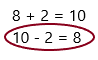Explanation:
The symbol “-” signifies subtraction. A subtraction process consists of three parts of numbers, namely minuend, subtrahend and difference. The number in a subtraction sentence from which we subtract another number is called a minuend, which means the minuend is the first number in a subtraction process.
Given 10 – 2 = 8
Here,
10 = Minuend
2 = Subtrahend
8 = Difference
And, “-” is the minus, i.e. the symbol for subtraction and “=” is the equal sign.

Question 3.
Circle the count on fact.
5 – 1 = 4
4 + 1 = 5Explanation:
Mental math strategy to add two numbers. We start with the biggest number of the numbers to add and count up to the second.
Count-on facts are numbers 0 – 9 that only add +1, +2, +3. When practising count-on start with the largest number and count up. For example 5+3. Say 5 and then count-on 6,7,8

Question 4.
Circle the count back fact.
8 – 2 = 6
6 + 2 = 8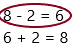A method of learning subtraction by counting backwards from the first number in the problem to get the answer.
We need to count backwards from 8:
8, 7, 6. This is how we count backwards.

“Finding Ten”
written by Mike Mason
This Take-Home Book belongs to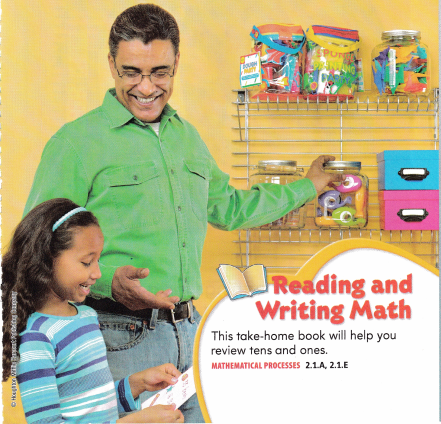Answer: This book belongs to review the ones and tens.
Which means addition and counting the things. And also choosing the things in a set and single.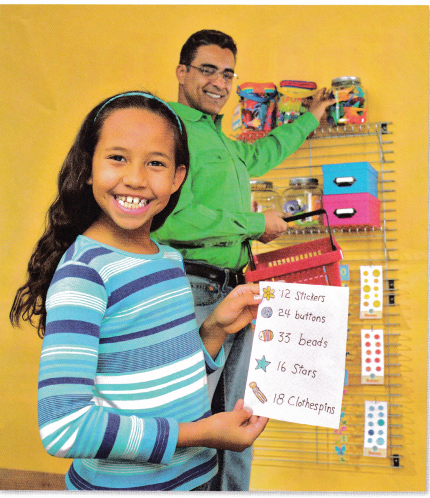Keri will make things for a craft fair. Keri has a list of things she needs to get for her crafts. Can you read her list?
In the list, she has 5 items for making a craft. They are:
12 stickers, 24 buttons, 33 beads, 16 stars, 18 clothespins.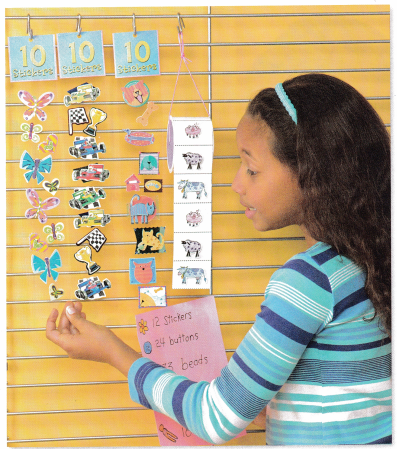Keri needs 12 stickers for her project. The stickers ore sold in sets of ten or one at a time. Circle the stickers you think Keri will buy.
Answer: 2 sets of six stickers.Explanation:
– The stickers were sold out that are in a set having with 10 stickers each.
– In the above diagram, circled a set of 6 stickers.
– Definitely, she will buy 2 sets of those stickers having each in 6.
– She needs 12 stickers so she buys 2 sets which is nothing but 2*6=12.
– Therefore, 12 stickers will be there.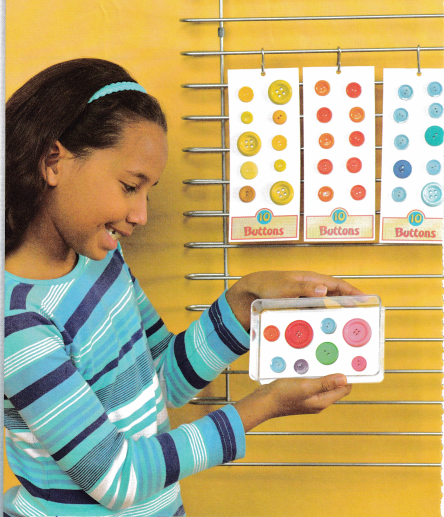Keri needs 24 buttons. The buttons are sold in sets of ten or as single buttons. Circle the buttons you think Keri will buy.
Answer: 2 sets of 10 buttons and 4 single buttons.Explanation:
– The buttons were sold in sets that are in a set having 10 buttons each.
– There are 8 single buttons.
– In the above diagram, circled 2 sets of 10 buttons and single buttons.
– She needs 24 buttons
– Definitely, she will buy 2 sets of those buttons having each in 10.
– She needs 24 buttons so she buys 2 sets which is nothing but 2*10=20.
– And remaining 4 buttons will be bought from single buttons.
– Now she has 20+4=24 buttons.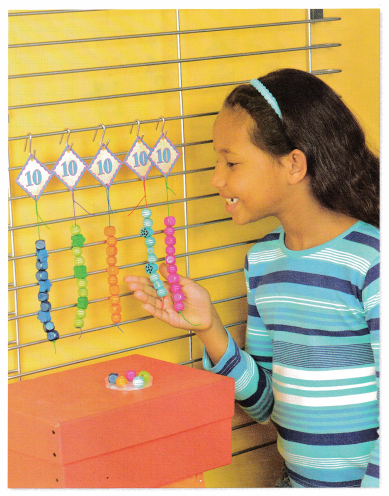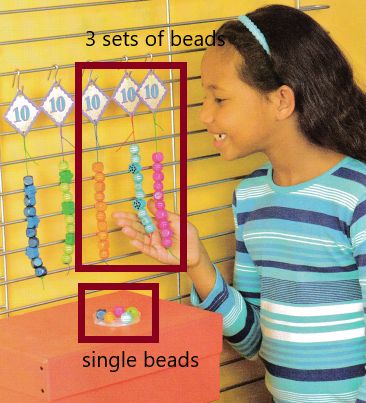Explanation:
– The buttons were sold in sets that are in a set having 10 beads each.
– There are 5 single beads.
– In the above diagram, circled 3 sets of 10 beads and single buttons.
– Definitely, she will buy 3 sets of those beads having each in 10.
– She needs 33 beads so she buys 3 sets which is nothing but 3*10=30.
– Now she has 30+3=33 beads.Keri needs 16 stars. Stars are sold in § sets of ten, or as single stars. Circle the stars you think Keri will buy.
Answer: 1 set of 10 stars and 6 single stars.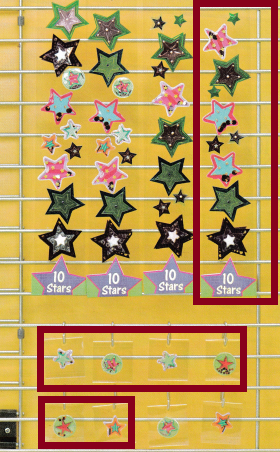Explanation:
– The buttons were sold in sets that are in a set having 10 stars each.
– There are 8 single stars overall.
– In the above diagram, circled 1 set of 10 stars and 6 single stars.
– She needs 16 stars.
– Definitely, she will buy 1 set of those stars having each in 10.
– Since she needs 16 stars so she buys 1 set which is nothing but 1*10=10.
– And the remaining 6 stars will be bought from single stars.
– Now she has 10+6=16 stars.Keri needs 18 clothespins. Which clothespins will she buy? Circle them.
Answer: She will buy a set having 10 clothespins and she buys a single clothespin present below the sets.Explanation:
She wants to buy 18 clothespins so she takes one set and 8 single pins.
In math sentence, we can say:
The number of pins in a set=10
The number pins in a single=9.
In that 9 pins, she will take 8 pins because she needs 18 pins. Already in a set 10 pins will be there. Now totally she will have:
10+8=18 clothespins.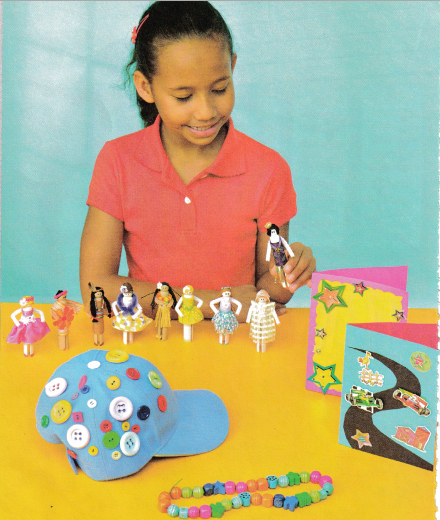When Keri got home she got busy! Look at the great things she made for the sale!
– She made greetings with 16 stars.
– She decorated a cap with 24 buttons.
– She made dolls with 18 clothespins.

Vocabulary Review
tens
ones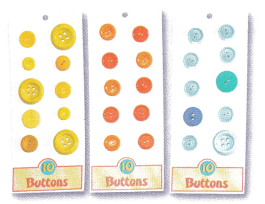There are 3 sets 10 ones
In each set, there are 1 tens.
3 sets of 10 ones mean 3*10=30 buttons.
1 ten in each set mean 1*10=10 buttons.
In each set, there are 10 buttons. Likewise, 3 sets are there so overall 30 buttons are there.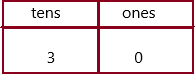This can be written as 30+0=30.

Place Value of 2-Digit Numbers

Use the picture to answer the questions.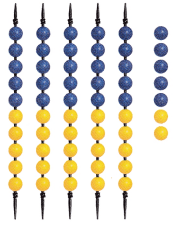Question 1.
How many sets of 10 beads are there?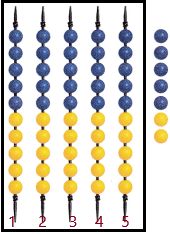Explanation:
The number of blue beads in each line=5
The number of yellow beads in each line=5
Likewise, 5 lines are there, each line having 10 beads.
Therefore, 5sets of 10 beads are present.

Question 2.
How many single beads are there?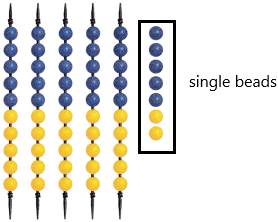Explanation:
The single beads are represented in the above diagram.
Count the beads which are single.
Therefore, 7 single beads are there.

Question 3.
How many beads are there in all?
Explanation:
The number of beads in each line=10
The number of lines=5
The total number of beads in sets=5*10=50
X=50+7
X=57
Therefore, 57 beads are there overall.
Question 4.
Explanation:
The number of beads in each set=10
Now the number of beads is present=X
X=20+3
X=23

Question 5.
Explanation:
The number of beads in each set=10